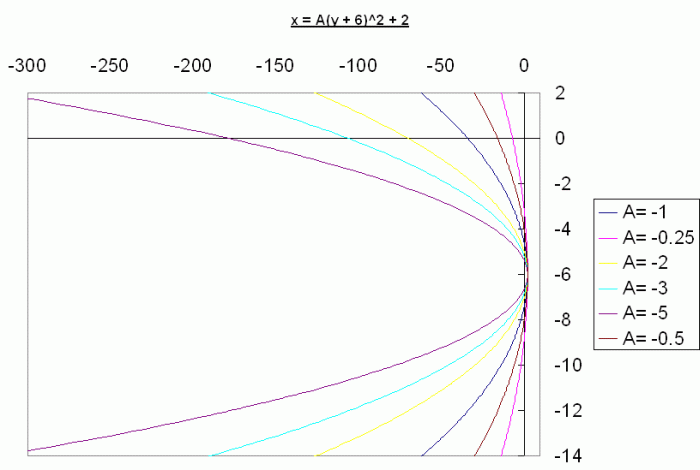SEARCH HOMEMath Central Quandaries & QueriesQuestion from Andy, a student: How do you write an equation of a parabola that opens to the left with a vertex of (2,-6)?Hi Andy.

The equation of a parabola opening up or down is y = A (x - h)2 + k, where (h, k) is the vertex of the parabola and A indicates direction and narrowness/wideness.

So the equation of a parabola opening right or left is x = A (y - k)2 + h. Since you are opening to the left, we know that the value of A is negative.

So we have x = A (y + 6)2 + 2, where we don't know much about A, except that it is negative.

Here are a few graphs of this equation with various negative values for A. You see what happens?Thus, the answer to your question is that there are many equations like this. You need to know more about the parabola to decide on a particular equation.

Stephen La Rocque. >Math Central is supported by the University of Regina and The Pacific Institute for the Mathematical Sciences.